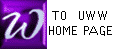FUNCTIONAL NOTATION (problem  )
and
COMPOSITE FUNCTIONS (i.e., CHAINS) (problem  )

   If f(x) = 3x+2 , and if g(x) = x2 - 1, then find the following:

 a] f(1) b] f(0) c] f(-1) d] f(x+1) e] f(x-1) f] f(3x)

g] f(3x+2)
h] f(-x)
i] f(x2)
j] f(f(x))
k] f(g(x))
 Extra problem f(f(f(x)))

 l] g(1) m] g(0) n] g(-1) o] g(x+1) p] g(x-1) q] g(3x)

r] g(-x)
s] g(x2)
t] g(x2-1)
u] g(f(x))
v] g(g(x))
 Extra problem g(x + h)

 The following exercizes practice skills used in THE CHAIN RULE from Calculus. Composite functions (or chains) have the form y(u(x)). In this situation we may call u or u(x) the "inner function", and we may call y or y(u) the "outer function". For the functions h(x) listed below, choose a u(x) and an y(u), so that their composition y(u(x)) is equal to the given h(x).
 Example: If h(x) = (2x + 1)2, choose u = 2x + 1 and y(u) = u2

a] h(x) = (x2 - 7x)6 h] h(x) = e-x
b] h(x) =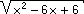i] h(x) =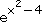c] h(x) = (3x + 2) - 4 j] h(x) =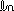(3x - 2)
 d] h(x) = 1    2x + 1
k] h(x) =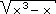e] h(x) = x l] h(x) =(ex)
 f] h(x) = 1     (x2 - 9)3
 m] h(x) = 1x
h(x) = e6x n] h(x) =(x)